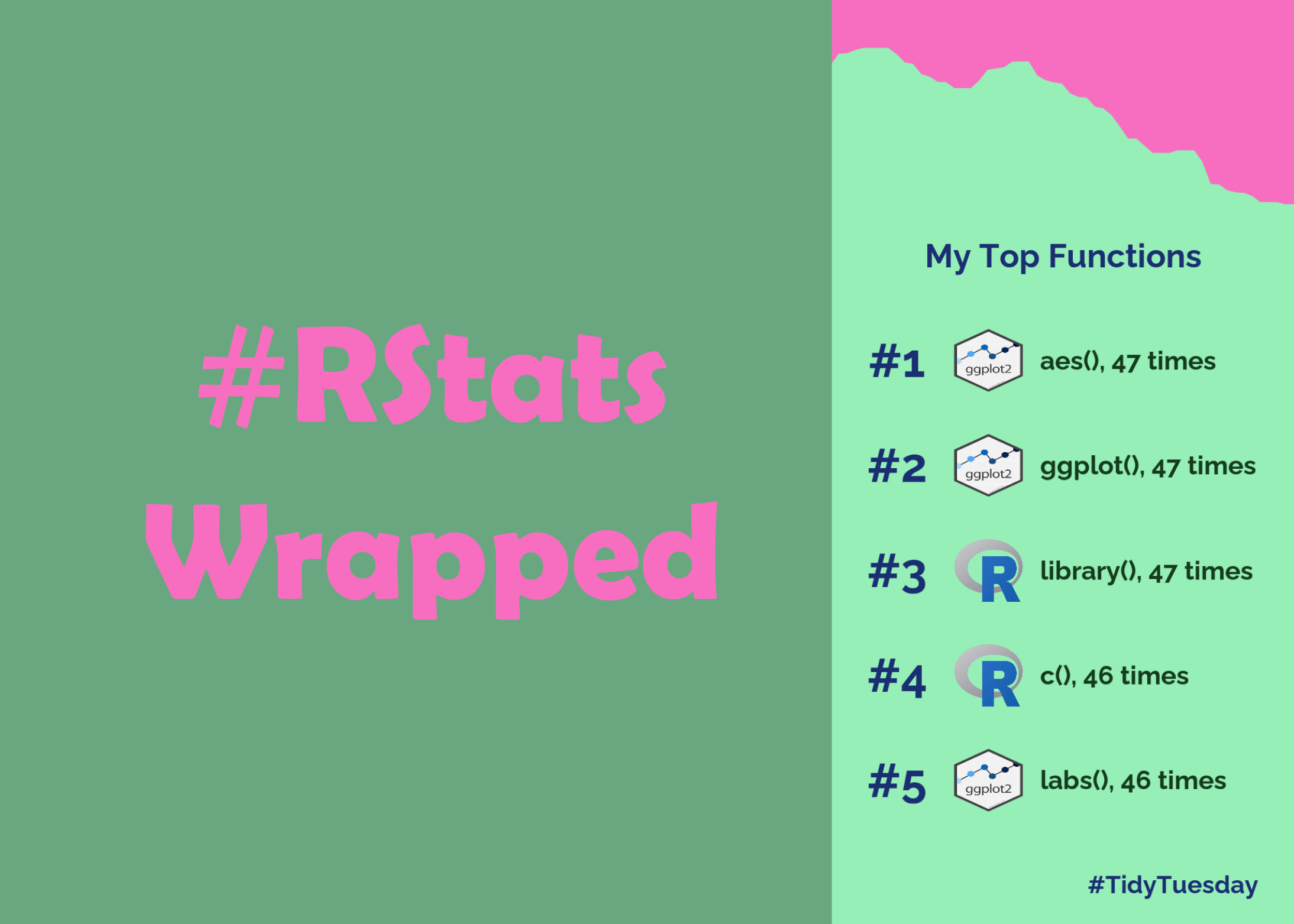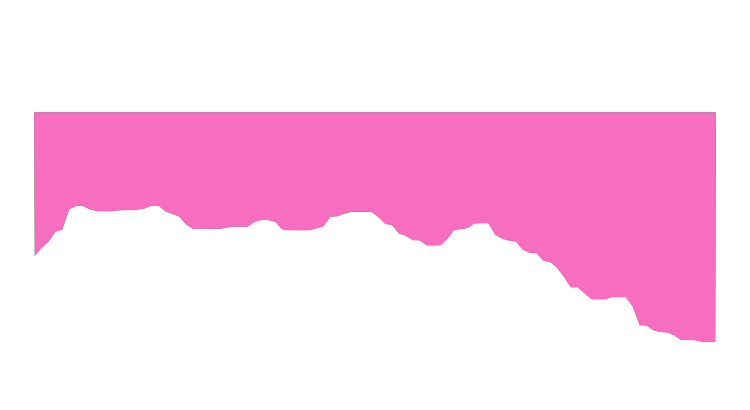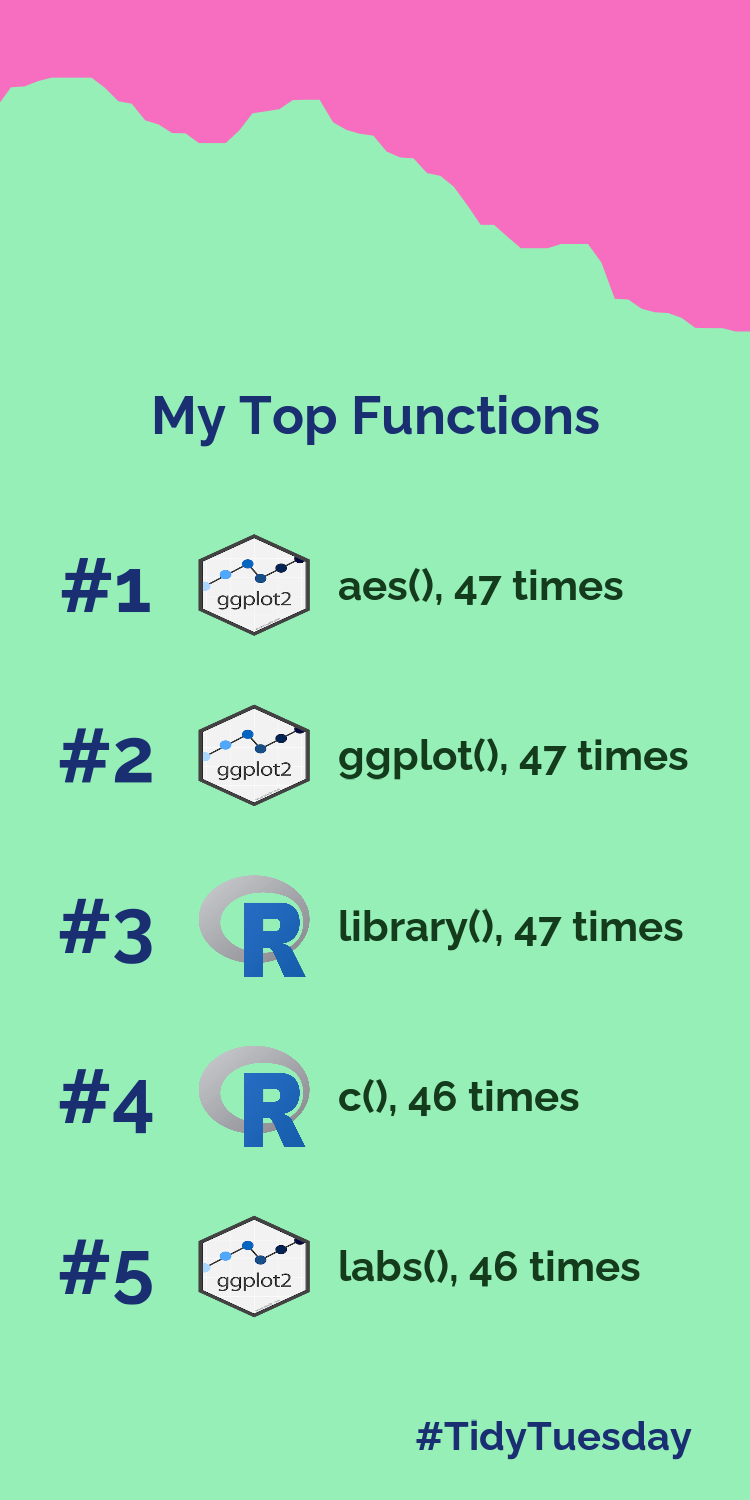Want to share your content on R-bloggers? click here if you have a blog, or here if you don't.

Around the beginning of December social media accounts start to fill with images of people’s Spotify Wrapped showing their most listened to songs. If you’re an #RStats user, you might also be interested in which functions you use most often. This blog post will show you how to create your own #RStats Wrapped!### Finding your most used functions

First of all, let’s load the packages you’re going to need:

```library(NCmisc)
library(janitor)
library(tidyverse)
library(patchwork)
library(showtext)
library(ggtextures)
```

Then choose which directory you want to look through for .R files. I’d recommend not saving the file you’re writing your analysis in the same directory, otherwise you’ll end up including half of the code you’re currently writing in your counts as well. You can then use `list.files()` to get a list of all the file names in that directory – make sure you set `recursive = TRUE` to get files in sub-folders.

```fpath <- glue::glue(here::here(), "/2022")
all_files <- list.files(path = fpath, recursive = TRUE)
```

Now let’s filter that list of files to only grab the .R files! Let’s also convert the vector of file names to a tibble so that you can use some {dplyr} functions to process it. You can find the file type using `str_extract()`, and then use `filter()` to select only those with `.R` extensions:

```to_search <- all_files %>%
as_tibble() %>%
mutate(filetype = str_extract(value, pattern = "\\..*")) %>%
filter(filetype == ".R") %>%
pull(value)
```

Now we actually get to the main part of getting our most used functions! The workhorse of this is `list.functions.in.file()` from {NCmisc}. It scans through a file and returns the functions used in a script. Note that this doesn’t return the number of times each function is used in a script, just which functions are used. This is why I exclusively looked at my #TidyTuesday scripts - the result will be how many weeks I used a function, rather than how many times I used a function. If you’re interested in the number of times a function is written in a script, you could use a combination of `utils::parse()` and `utils::getParseData()` to do this (which `list.functions.in.file()` is a wrapper around).

You can then use {purrr} to map over all of the files in our `to_search` vector and apply the `list.functions.in.file()` to each file:

```all_functions <- map(.x = to_search, .f = ~ list.functions.in.file(.x))
```

For me, the first result looks something like this:

```\$`character(0)`
 "draw_plot"     "geom_textpath" "ggdraw"

\$`package:base`
 "cos"        "data.frame" "library"    "readLines"  "seq"        "sin"

\$`package:ggplot2`
 "aes"           "coord_equal"   "element_blank" "element_rect"  "element_text"  "ggplot"
 "labs"          "margin"        "theme"         "theme_void"
```

Depending on how many files you have, this may take a little while to run. If you’re planning to do this analysis in multiple stages, you might want to save this object so you can read it in quicker later on. You’ll also notice that the package names are returned, so if you want to look for your most used packages instead of most used functions, you can use the same function!

Now, you can process the data and select your top five packages:

```func_data <- unlist(all_functions) %>%
unname() %>%
tabyl() %>%
as_tibble() %>%
rename(func = ".") %>%
slice_max(n, n = 5, with_ties = FALSE)
```

Here, the `tabyl()` functon from {janitor} package is counting the number of scripts that each function was used in. Setting `with_ties = FALSE` ensures that you get exactly 5 results returned.

```# A tibble: 5 × 3
func        n percent
<chr>   <int>   <dbl>
1 aes        47  0.0284
2 ggplot     47  0.0284
3 library    47  0.0284
4 c          46  0.0278
5 labs       46  0.0278
```

Now, there comes a bit more manual work - you need to find some images for each function or package in the list! Here, I’m assuming that you know which package a function comes from and can (hopefully) look up the hex sticker for the package!

```imgs <- tibble(img = c("https://ggplot2.tidyverse.org/logo.png",
"https://ggplot2.tidyverse.org/logo.png",
"https://www.r-project.org/logo/Rlogo.png",
"https://www.r-project.org/logo/Rlogo.png",
"https://ggplot2.tidyverse.org/logo.png"))
```

Now comes the fun part - recreating the graphic! Here, you’ll mainly use {ggplot2} alongside a little bit of help from {patchwork} and {ggtextures}! First let’s choose and load a font using {showtext}:

```font_add_google("Raleway", "raleway")
showtext_auto()
```

Create the main graphic:

```p <- ggplot() +
# add text with the numbers 1 to 5
geom_text(data = data.frame(),
mapping = aes(x = rep(1, 5),
y = 1:5,
label = paste0("#", 1:5)),
colour = "#1a2e72",
size = 20,
fontface = "bold",
family = "raleway") +
# add text with the names of the functions, and the number of times its used
geom_text(data = func_data,
mapping = aes(x = rep(2.25, 5),
y = 1:5,
label = paste0(func, "(), ", n, " times")),
colour = "#143b1c",
hjust = 0,
size = 11,
fontface = "bold",
family = "raleway") +
# add images for each package
geom_textured_rect(data = imgs,
aes(xmin = rep(1.5, 5), xmax = rep(2.1, 5),
ymax = 1:5-0.3, ymin = 1:5+0.3, image = img),
lty = "blank",
fill="transparent",
nrow = 1,
ncol = 1,
img_width = unit(1, "null"),
img_height = unit(1, "null"),
position = "identity")  +
geom_text(data = data.frame(),
aes(x = 2.45, y = 0, label = "My Top Functions"),
colour = "#1a2e72",
fontface = "bold",
hjust = 0.5,
size = 14,
family = "raleway") +
# set axis limits and reverse y axis
scale_x_continuous(limits = c(0.9, 4)) +
scale_y_reverse(limits = c(5.5, -0.2)) +
labs(caption = "#TidyTuesday") +
# set the theme
theme_void() +
theme(plot.background = element_rect(fill = "#96efb7", colour = "#96efb7"),
panel.background = element_rect(fill = "#96efb7", colour = "#96efb7"),
plot.margin = margin(40, 15, 10, 15),
plot.caption = element_text(colour = "#1a2e72",
margin = margin(t = 15),
face = "bold",
hjust = 1,
size = 30,
family = "raleway"))
```

You may have used `geom_image()` from {ggimage} to add images to a plot before - I had a few issues with this initially. It seems that `geom_image()` re-scales images to the same aspect ratio as the plotting area which doesn’t really work here. Instead I used `geom_textured_rect()` from {ggtextures} which allows you to specify a width and height for your image.When you’re making graphics in RStudio, I’d recommend using {camcorder} or {ggview} to show your plot in the Viewer tab of RStudio using the same dpi you’ll end up saving your image with using `ggsave()`. This helps to avoid issues with fonts not re-scaling when saving your image.

Most of the Spotify Wrapped graphics also have some sort of patterned background. You could use a background image and stick your {ggplot2} graphic on top with {patchwork} or {cowplot}, or you could try to make a background using {ggplot2}! I used `geom_area()` to create a coloured shape:

```set.seed(2022)
curve1 <- tibble(x = 1:100) %>%
mutate(y = 20 + smooth(cumsum(rnorm(100))))
inset1 <- ggplot(data = curve1,
aes(x = x, y = y)) +
geom_area(fill = "#f76ec0") +
theme_void() +
coord_fixed() +
scale_y_reverse()
```Now, you can overlay the coloured area using {patchwork}:

```p + inset_element(inset1, left = -1, right = 1.2, bottom = 0, top = 2.2, align_to = "full") &
theme(plot.background = element_rect(fill = "#96efb7", colour = "#96efb7"),
panel.background = element_rect(fill = "#96efb7", colour = "#96efb7"),
plot.margin = margin(40, 7, 5, 7),
plot.caption = element_text(colour = "#1a2e72",
margin = margin(t = 5),
face = "bold",
hjust = 1,
size = 30))
```

It took a little bit of trial and error to get the positioning of the inset element and the margins to get something I was happy with - if you generate a different inset element, you might want to choose different values!Finally, save your image using `ggsave()`:

```ggsave("tidytuesday_wrapped.png", width = 2.5, height = 5, units = "in")
```

I’d like to do a little more work on the background of this, and maybe consider calculating the number of uses of a function instead of number of scripts it’s used in. But, hopefully now you can create your own #RStats Wrapped! Which functions do you use most often?